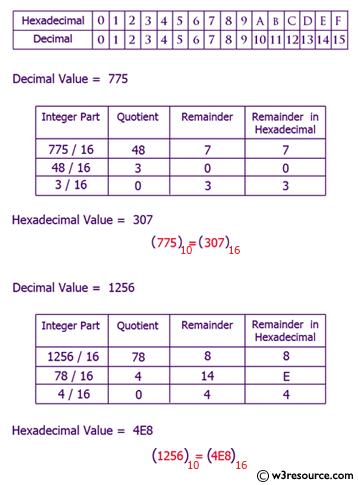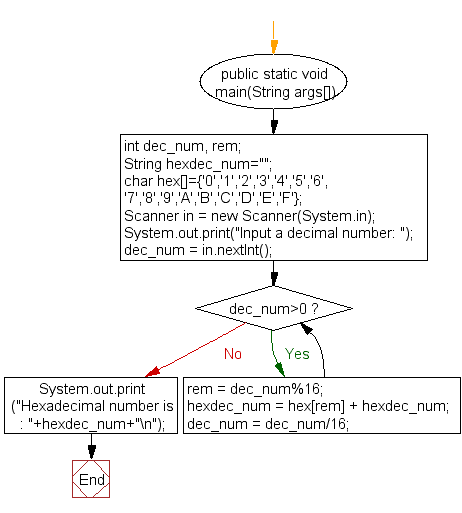﻿ Java exercises: Convert a decimal number to hexadecimal number - w3resource# Java Exercises: Convert a decimal number to hexadecimal number

## Java Basic: Exercise-20 with Solution

Write a Java program to convert a decimal number to hexadecimal number.

Decimal number: The decimal numeral system is the standard system for denoting integer and non-integer numbers. It is also called base-ten positional numeral system.

Hexadecimal number: Hexadecimal is a positional numeral system with a radix, or base, of 16. It uses sixteen distinct symbols, most often the symbols 0-9 to represent values zero to nine, and A, B, C, D, E, F (or alternatively a, b, c, d, e, f) to represent values ten to fifteen.

Test Data:
Input a decimal number: 15

Pictorial Presentation: Decimal to Hexadecimal numberSample Solution:

Java Code:

``````import java.util.Scanner;
public class Exercise20 {
public static void main(String args[])
{
int dec_num, rem;
String hexdec_num="";

char hex[]={'0','1','2','3','4','5','6','7','8','9','A','B','C','D','E','F'};

Scanner in = new Scanner(System.in);

System.out.print("Input a decimal number: ");
dec_num = in.nextInt();

while(dec_num>0)
{
rem = dec_num%16;
hexdec_num = hex[rem] + hexdec_num;
dec_num = dec_num/16;
}
}
}
```
```

Sample Output:

```Input a decimal number: 15
```

Flowchart:Java Code Editor:

What is the difficulty level of this exercise?

Test your Programming skills with w3resource's quiz.

﻿

## Java: Tips of the Day

getEnumMap

Converts to enum to Map where key is the name and value is Enum itself.

```public static <E extends Enum<E>> Map<String, E> getEnumMap(final Class<E> enumClass) {
return Arrays.stream(enumClass.getEnumConstants())
.collect(Collectors.toMap(Enum::name, Function.identity()));
}
```

Ref: https://bit.ly/3xXcFZt